# Olympiad Test: Figure Matrix - 2

## 15 Questions MCQ Test Mathematical Olympiad Class 8 | Olympiad Test: Figure Matrix - 2

Description
Attempt Olympiad Test: Figure Matrix - 2 | 15 questions in 30 minutes | Mock test for Class 8 preparation | Free important questions MCQ to study Mathematical Olympiad Class 8 for Class 8 Exam | Download free PDF with solutions
QUESTION: 1

Solution:
QUESTION: 2

Solution:
QUESTION: 3

### Direction: In the following questions, study the given matrix, and find the correct option for the question mark form the given four options.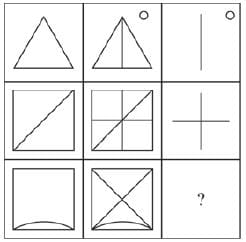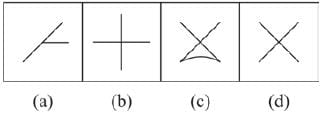Solution:

In each row, the 2nd figure is obtained by shading one of the four parts of the first figure.

QUESTION: 4

Direction: In the following questions, study the given matrix, and find the correct option for the question mark form the given four options.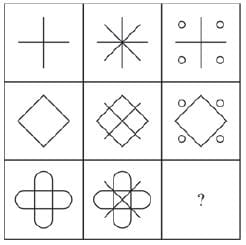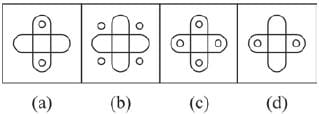Solution:

The 3rd figure in each row consists of parts which are not common in first two figures.

QUESTION: 5

Direction: In the following questions, study the given matrix, and find the correct option for the question mark form the given four options.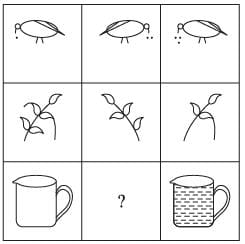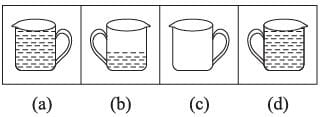Solution:
QUESTION: 6

Direction: In the following questions, study the given matrix, and find the correct option for the question mark form the given four options.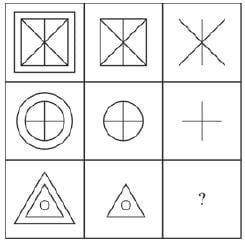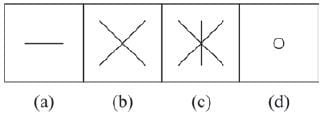Solution:
QUESTION: 7

Direction: In the following questions, study the given matrix, and find the correct option for the question mark form the given four options.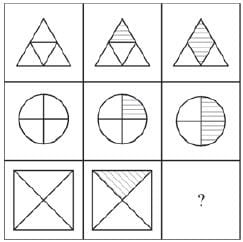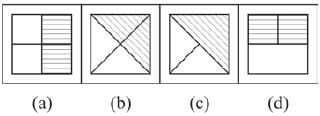Solution:
QUESTION: 8

Direction: In the following questions, study the given matrix, and find the correct option for the question mark form the given four options.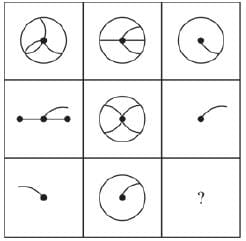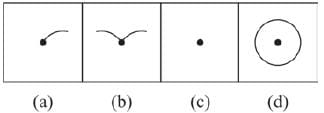Solution:
QUESTION: 9

Direction: In the following questions, study the given matrix, and find the correct option for the question mark form the given four options.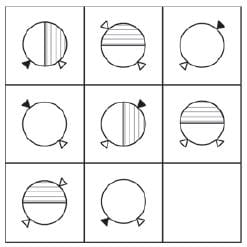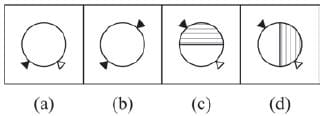Solution:
QUESTION: 10

Direction: In the following questions, study the given matrix, and find the correct option for the question mark form the given four options.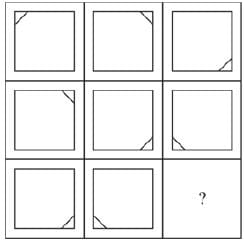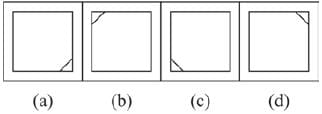Solution:
QUESTION: 11

Direction: In the following questions, study the given matrix, and find the correct option for the question mark form the given four options.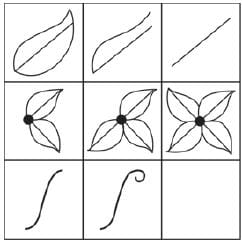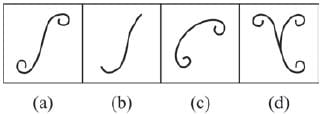Solution:
QUESTION: 12

Direction: In the following questions, study the given matrix, and find the correct option for the question mark form the given four options.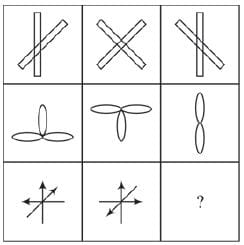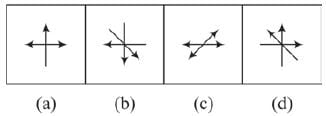Solution:
QUESTION: 13

Direction: In the following questions, study the given matrix, and find the correct option for the question mark form the given four options.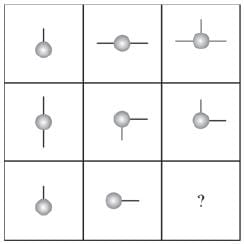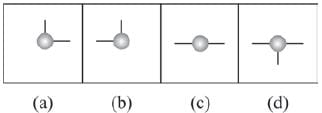Solution:
QUESTION: 14

Direction: In the following questions, study the given matrix, and find the correct option for the question mark form the given four options.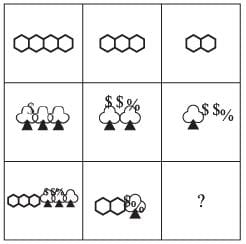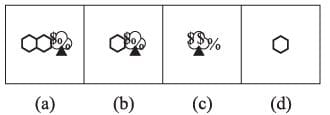Solution:
QUESTION: 15

Direction: In the following questions, study the given matrix, and find the correct option for the question mark form the given four options.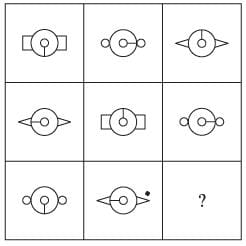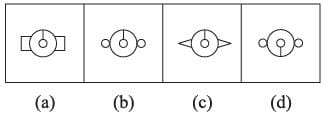Solution:Use Code STAYHOME200 and get INR 200 additional OFF Use Coupon Code